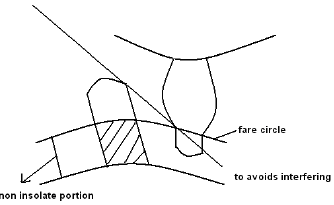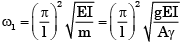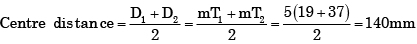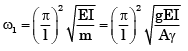Courses

# Critical Speeds Or Whirling Of Shaft And Gear Train And Gear Design - MCQ Test 1

## 20 Questions MCQ Test Mock Test Series - Mechanical Engineering (ME) for GATE 2020 | Critical Speeds Or Whirling Of Shaft And Gear Train And Gear Design - MCQ Test 1

Description
This mock test of Critical Speeds Or Whirling Of Shaft And Gear Train And Gear Design - MCQ Test 1 for Mechanical Engineering helps you for every Mechanical Engineering entrance exam. This contains 20 Multiple Choice Questions for Mechanical Engineering Critical Speeds Or Whirling Of Shaft And Gear Train And Gear Design - MCQ Test 1 (mcq) to study with solutions a complete question bank. The solved questions answers in this Critical Speeds Or Whirling Of Shaft And Gear Train And Gear Design - MCQ Test 1 quiz give you a good mix of easy questions and tough questions. Mechanical Engineering students definitely take this Critical Speeds Or Whirling Of Shaft And Gear Train And Gear Design - MCQ Test 1 exercise for a better result in the exam. You can find other Critical Speeds Or Whirling Of Shaft And Gear Train And Gear Design - MCQ Test 1 extra questions, long questions & short questions for Mechanical Engineering on EduRev as well by searching above.
QUESTION: 1

### In vibration isolation system, if ω/ωn, then the phase difference between the transmitted force and the disturbing force is

Solution:

In Vibration-isolation system, If the phase difference between transmitted force and the disturbing force is 180°C, then ω/ωn = 1.

QUESTION: 2

Solution:
QUESTION: 3

### Which one of the following causes the whirling of shafts?

Solution:
QUESTION: 4

In spur gears, the circle on which the involute is generated is called the

Solution:
QUESTION: 5

Rotating shafts tend of vibrate violently at whirling speeds because

Solution:
QUESTION: 6

Tooth interference in an external in volute spur gear pair can be reduced by

Solution:There are several ways to avoid interfering:
i. Increase number of gear teeth
ii. Modified involutes
iv. Increased centre distance

QUESTION: 7

Critical speed of a shaft with a disc supported in between is equal to the natural frequency of the system in

Solution:
QUESTION: 8

The minimum number of teeth on the pinion to operate without interference in standard full height involute teeth gear mechanism with 20° pressure angle is

Solution:
QUESTION: 9

The critical speed of a rotating shaft depends upon

Solution:QUESTION: 10

Interference in a pair of gears is avoided, if the addendum circles of both the gears intersect common tangent to the base circles within the points of tangency.

Solution:
QUESTION: 11

A shaft has two heavy rotors mounted on it. The transverse natural frequencies, considering each of the rotor separately, are 100 Hz and 200 Hz respectively. The lowest critical speed is

Solution:
QUESTION: 12

A spur gear has a module of 3 mm, number of teeth 16, a face width of 36 mm and a pressure angle of 20°. It is transmitting a power of 3 kW at 20 rev/s. Taking a velocity factor of 1.5, and a form factor of 0.3, the stress in the gear tooth is about

Solution:
QUESTION: 13

A shaft carries a weight W at the centre. The CG of the weight is displaced by an amount e from the axis of the rotation. If y is the additional displacement of the CG from the axis of rotation due to the centrifugal force, then the ratio of y to e (where ωc, is the critical speed of shaft and w is the angular speed of shaft) is given by

Solution:
QUESTION: 14

Twenty degree full depth involute profiled 19-tooth pinion and 37-tooth gear are in mesh. If the module is 5 mm, the centre distance between the gear pair will be

Solution:QUESTION: 15

The critical speed of a shaft is affected by the

Solution:QUESTION: 16

A 20o full depth involute spur pinion of 4 mm module and 21 teeth is to transmit 15 kW at 960 rpm. Its face width is 25 mm.

Q. The tangential force transmitted (in N) is

Solution:
QUESTION: 17

A 20o full depth involute spur pinion of 4 mm module and 21 teeth is to transmit 15 kW at 960 rpm. Its face width is 25 mm.

Q.

Given that the tooth geometry factor is 0.32 and the combined effect of dynamic load and allied factors intensifying the stress is 1.5; the minimum allowable stress (in MPa) for the gear material is

Solution:
QUESTION: 18

Number of teeth on a wheel per unit of its pitch diameter is called

Solution:
QUESTION: 19

A gear set has a pinion with 20 teeth and a gear with 40 teeth. The pinion runs at 30 rev/s and transmits a power of 20 kW. The teeth are on the 20c full-depth system and have a module of 5 mm. The length of the line of action is 19 mm.

Q. The center distance for the above gear set in mm is​

Solution:
QUESTION: 20

A gear set has a opinion with 20 teeth and a gear with 40 teeth. The pinion runs at 0 rev/s and transmits a power of 20 kW. The teeth are on the 20° full –depth system and have module of 5 mm. The length of the line of action is 19 mm.

Q.

The contact ratio of the contacting tooth

Solution: